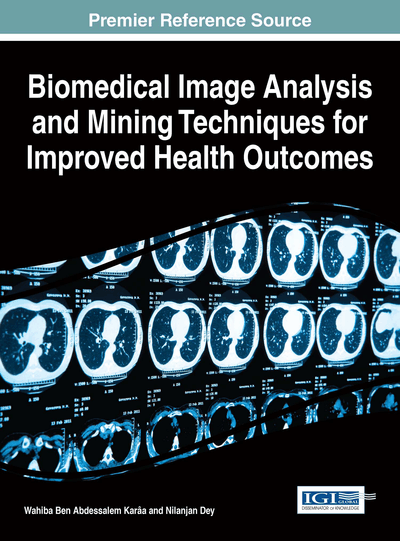# Compressed Sensing and Its Application in CT and EEG

Sajib Saha (The University of New South Wales, Australia) and Murat Tahtali (The University of New South Wales, Australia)
DOI: 10.4018/978-1-4666-8811-7.ch006

## Abstract

Compressed sensing, also known as compressive sampling is a new technique being rapidly developed over the last few years. The theory states that when some prior information about the signal is available and appropriately incorporated into the signal reconstruction procedure, a signal can be accurately reconstructed even if the Shannon/ Nyquest sampling requirement is violated. The key idea of compressed sensing is to recover a sparse signal from very few non-adaptive, linear measurements by optimization technique. Following the discovery by Donoho in (2006), that sparsity could enable exact solution of ill-posed problems under certain conditions, there has been a tremendous growth on efficient application of sparsity constraints for solving ill-posed problems. The theoretical foundation of compressed sensing has already become a key concept in various areas of applied mathematics, computer science, and electrical engineering. In this chapter we will detail the application of compressed sensing in X-ray computed tomography (CT) and Electroencephalography. Starting from the very basic principles we will provide theoretical justifications on why and how sparsity prior is used in CT and in EEG.
Chapter Preview
Top

## Introduction To Compressed Sensing

Compressive sensing (CS) is a novel sampling paradigm that allows the sampling of signals in a much more efficient way than the established Nyquist-Shannon sampling theorem. In 1949, Shannon (1998) presented his famous proof that, if a function f(t) contains no frequencies higher than W cps (cycles per second), it is completely determined by giving its ordinates at a series of points spaced at the critical sampling interval of 1/2W, to which he referred as the Nyquist interval, in recognition of Nyquist’s discovery of the fundamental importance of this interval in connection with telegraphy (Meijering, 2002).Traditional signal processing techniques generally sample data uniformly at the Nyquist rate, however compressed sensing theory states that certain signals can be recovered from fewer samples than required in the Nyquist-Shannon paradigm. David L. Donoho et al. in (2010) say “The sampling theorem of Shannon-Nyquist-Kotelnikov-Whittaker has been of tremendous importance in engineering theory and practice. Straightforward and precise, it sets forth the number of measurements required to reconstruct any band limited signal. However, the sampling theorem is wrong! Not literally wrong, but psychologically wrong. More precisely, it engender(s) the psychological expectation that we need very large numbers of samples in situations where we need very few.”

Contrary to traditional Nyquist-Shannon paradigm, the CS paradigm, banking on finding sparse solutions to under-determined linear systems, can reconstruct signals from far fewer samples than the Nyquist-Shannon sampling rate. This recovery is exact if the signal being sensed has a low information rate (means it is sparse in the original or in some transform domain).

The key idea of compressed sensing is to recover a sparse signal from very few non-adaptive, linear measurements by optimization techniques (Eldar et al., 2012). This development – the problem of sparse recovery – can in fact be traced back to earlier papers from the 90s such as (Donoho et al., 1989), and later the prominent papers by Donoho and Huo (Donoho et al., 2001) and Donoho and Elad (Donoho et al., 2003). Following the discovery that sparsity could enable the exact solution of ill-posed problems under certain conditions (Donoho, 2006; Candes et al., 2007), there has been a tremendous growth on efficient application of sparsity constraints for solving ill-posed problems (Saha et al., 2014b). The theoretical foundation of compressed sensing has already become a key concept in various areas of applied mathematics, computer science, and electrical engineering. It is worth mentioning that previously, when the fundamental papers introducing compressed sensing (Donoho et al., 2001; Donoho et al. 2003) were published, the term “compressed sensing” was initially utilized for random sensing matrices, since those allow for a minimal number of non-adaptive, linear measurements (Eldar et al., 2012). Nowadays, the term “compressed sensing” is more and more often used interchangeably with “sparse recovery” in general.

### Mathematical Formulation of the Problem

To state the problem mathematically precisely, let X represents the signal to be sensed, then the compressed sensing problem can be formulated as follows:

Y = AX. (1)

Where A is the m×n measurement matrix andmeasurement vector. Under conventional sensing paradigm m must be at least equal to n (Saad et al., 2013). However CS states that m can be far less than n provided the signal is sparse (accurate reconstruction) or nearly sparse/compressible (approximate reconstruction) in original or some transform domain.

A nonlinear algorithm is used in CS to recover X, with the prior assumption that X itself is sparse, i.e. it has very few non-zero coefficients in the sense that ||X||0:=#{i: Xi ≠ 0} is small, or that there exists an orthogonal basis or frame ψ such that X=ΨS with S being sparse. When X is sparse in the ψ domain, the compressed sensing problem in (1) can be reformulated as follows:

Y=A ψ S. (2)

It is quite intuitive to solve X with the knowledge of Y by solving

(L0) minX||X||0 subject to Y=AX.

This algorithm requires combinatorial search and is NP-hard (Edoardo et al., 1998; Muthukrishnan, 2005). Encouragingly, it has been shown in the fundamental paper (Chen et al. 1998) that, in many cases, it is possible to estimate the l0-norm by solving the following l1-norm optimization problem

(L1) minX||X||1 subject to Y=AX.

Now the general question when ‘l0 = l1’ holds the key to compressed sensing.

## Complete Chapter List

Search this Book:
Reset